Units - Definition with Examples

The Complete K-5 Math Learning Program Built for Your Child

• 30 Million Kids

Loved by kids and parent worldwide

• 50,000 Schools

Trusted by teachers across schools

• Comprehensive Curriculum

Aligned to Common Core

Unit

Units are the tools to measure and compare different things. Comparison becomes easy when all the units for the measurement are the same.  Different units can be classified depending on their use. A few of the units can be classified as:

Units to measure the length

The units of measuring length are millimeter (mm), centimeter (cm), meter (m) and kilometer (km).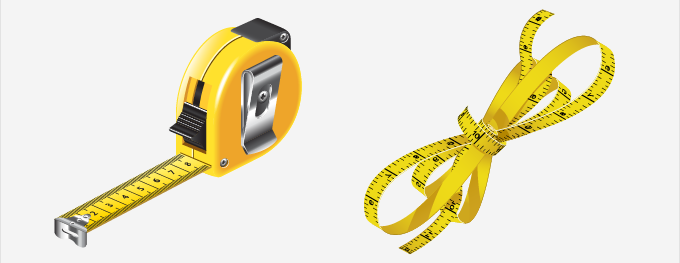mm and cm

Fact:

1 cm = 10 mm

Conversion:

cm → mm: Multiply by 10

mm → cm: Divide by 10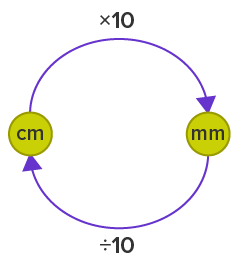cm and m

Fact:

1 m = 100 cm

Conversion:

m → cm:  Multiply by 100

c→ mm: Divide by 100

m and km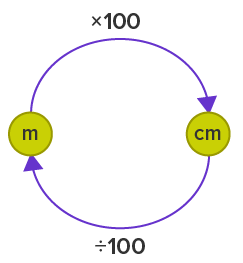Fact:

1 km = 1000 m

Conversion:

km→m: Multiply by 1000

mk→m: Divide by 1000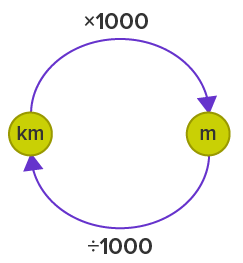Units to measure the weight

The units of measuring weight are kilogram (kg) and gram (g).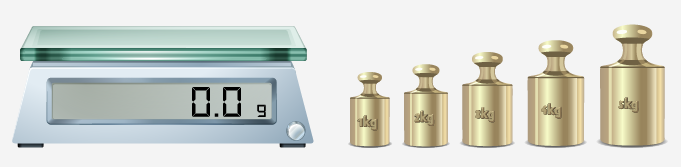kg and g

Fact:

1 kg = 1000 g

Conversion: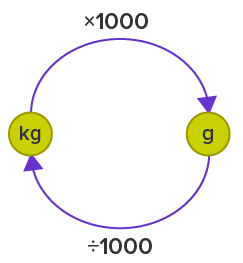kg→g: Multiply by 1000

g→kg: Divide by 1000

Units to measure the volume

The units of measuring volume are milliliter (ml) and liter (L).ml and L

Fact:

1 L = 1000 ml

Conversion:

L→ml: Multiply by 1000

ml→L: Divide by 1000

Conversion Examples:

Example 1: Do the following conversions.

(i) 400 cm to meters.

Solution: We know that to convert from centimeters to a meter, we divide by a 100.

Therefore, 400 cm=400 ÷100=4 m

(ii) 3 kg to grams.

Solution: We know that to convert from kilograms to grams, we multiply by 1000.

Therefore, 3 kg=3×1000=3000 g

(iii) 1.5 L to ml

Solution: We know that to convert from liters to milliliters, we multiply by 1000.

Therefore, 1.5 L=1.5×1000=1500ml

Example 3: Lucy, Eve, and Ragnar are comparing their heights. Lucy says that her height is 1 m, Eve says that her height is 900 mm and Ragnar says that his height is 97cm. Who is the tallest and the shortest person in height?

Solution:

Height of Lucy = 1 m

Height of Eve = 900 mm

Height of Ragnar = 97cm.

To compare their heights, we need to change them into the same unit.

Height of Lucy = 1 m = 1 × 100 = 100 cm

Height of Eve = 900 mm = 900 ÷ 10 = 90 cm

Height of Ragnar = 97 cm

Clearly, Lucy is the tallest and Eve is the shortest of all three.

 Fun Fact-  Ancient men didn’t use units to measure the length. Rather, they used their hand to measure the length which is now known as “hand-span”.

Won Numerous Awards & Honors